# The Augmented Truncated Dodecahedron

The augmented truncated dodecahedron is the 68th Johnson solid, or J68 for short. It has 42 faces (11 decagons, 1 pentagon, 5 squares, and 25 triangles), 105 edges, and 65 vertices. It is constructed by augmenting a truncated dodecahedron with a pentagonal cupola (J5).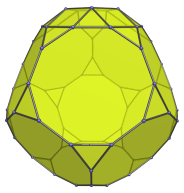Adding a second augment on the opposite decagon produces the parabiaugmented truncated dodecahedron (J69). Placing the second augment on a non-adjacent decagon that isn't the opposite one produces the metabiaugmented truncated dodecahedron (J70). The latter can take a third augment, producing the triaugmented truncated dodecahedron (J71).

## Projections

Here are some views of the augmented truncated dodecahedron from various angles:

Projection Description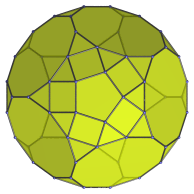Top view.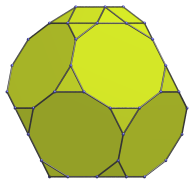Front view.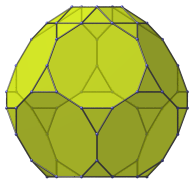Diagonal side view.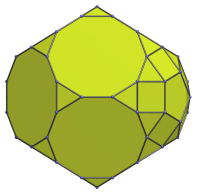Oblique view centered on decagon-decagon edge.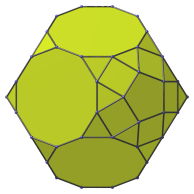Oblique view centered on decagon-triangle edge.

## Coordinates

The Cartesian coordinates of the augmented truncated dodecahedron with edge length 2 are even permutations of coordinate and all changes of sign of:

• (1, 0, 1+3φ)
• 2, 1, 2φ2)
• (2φ, φ2, φ3)

along with:

• ((13φ+1)/5, ±1, (6φ+12)/5)
• ((13φ+6)/5, ±φ, (φ+12)/5)
• ((18φ+1)/5, 0, (φ+7)/5)

where φ=(1+√5)/2 is the Golden Ratio.

Last updated 18 Jun 2019.